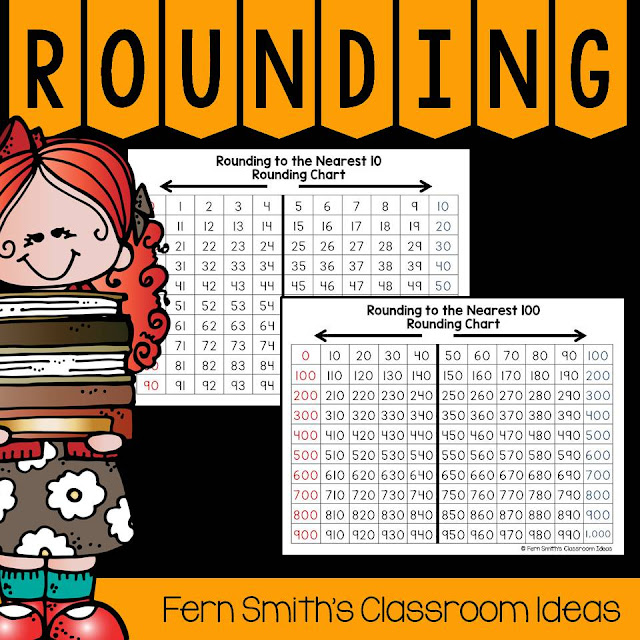### Are You Getting Ready to Start Teaching Rounding to Estimate Differences?If you are teaching Rounding to Estimate the Difference, I've got some great lessons, tips, resources and freebies for YOU!

A quick way to estimate the difference of two numbers is to round each number and then subtract the rounded numbers. {See this previous post for help on rounding.} More often than naught, this won't be the exact answer but it may be close enough for some purposes. Students need this mental math not only for future math classes and math procedures, but it is also an excellent life skill. Do you have enough money for all your groceries? Did the cashier give you the right amount of change back? When you relate math to MONEY, even eight year old ears perk up! Problem solving extends to all aspects of their world.Once your students have a strong foundation of rounding, you can move on to estimating the difference. Estimation is a powerful mathematical mental tool to be used both in solving problems and in checking the answer to see if it is reasonable.

Tips to introduce your lesson...
How to estimate a difference by rounding:
• Round each number in the problem.
• Subtract the rounded numbers together, mental math is even better.
More reasons WHY you would use rounding to estimate the difference:
• Check to see if you have about enough money to pay for your meal at restaurant before you order. Lobster or hamburger?
• Get a ball park idea to double check your answer to a problem. Is 300 an intelligent answer for 992 - 682?

Back to Top